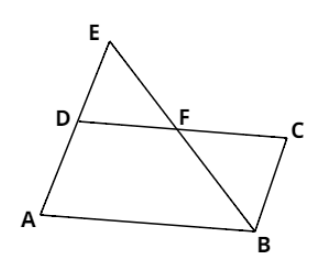Courses
Courses for Kids
Free study material
Free LIVE classes
MoreLIVE
Join Vedantu’s FREE Mastercalss

# E is a point on the side AD produced of a parallelogram ABCD and BE intersects CD at F. Show that $\Delta ABE \sim \Delta CFB$.Verified
360k+ views
Hint: Analyze the situation with a diagram. Use the properties that the opposite angles of a parallelogram are equal, its opposite sides are parallel. And the property that two angles made by the same line with two parallel lines are equal.Consider the parallelogram ABCD above. AD is produced up to E and BE intersects CD at F.

If we compare two triangles, $\Delta ABE$ and $\Delta CFB$:
$\Rightarrow \angle BAE = \angle BCF{\text{ }}\left[ {\therefore {\text{ Opposite angles of a parallelogram are equal}}} \right]$
$\Rightarrow \angle ABE = \angle CFB{\text{ }}\left[ {{\text{Angles made by the same line segment BE with parallel lines AB and CD}}} \right]$$\Rightarrow \angle AEB = \angle CBF{\text{ }}\left[ {{\text{Angles made by the same line segment BC with parallel lines AE and CD}}} \right]$
Since all the angles of triangles ABE and CFB are the same, we can say that the triangles are similar.

So, we have:
$\Rightarrow \Delta ABE \sim \Delta CFB$

This is the required proof.

Note: If two triangles are similar then the ratios of their corresponding sides are the same. For example, in above triangles ($\Delta ABE$ and $\Delta CFB$), since both the triangles are similar so we have:
$\Rightarrow \dfrac{{AB}}{{CF}} = \dfrac{{AE}}{{CB}} = \dfrac{{BE}}{{FB}}$
Last updated date: 19th Sep 2023
Total views: 360k
Views today: 7.60k